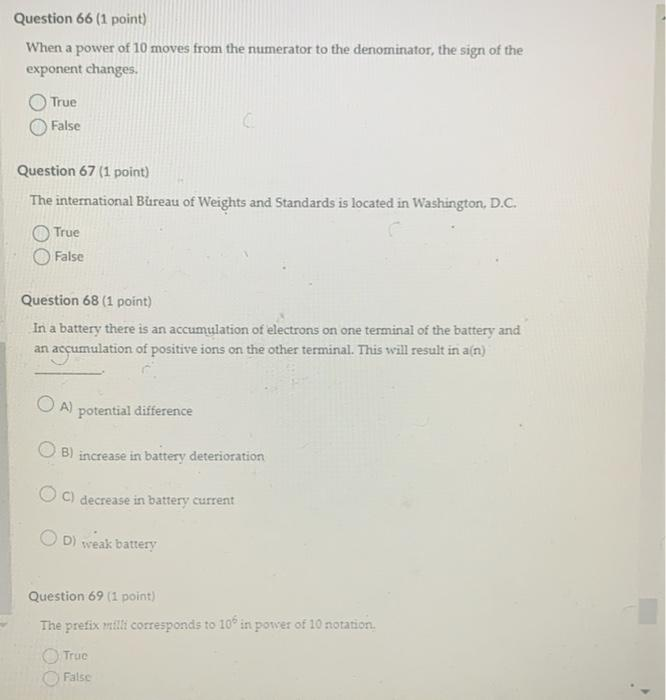Home / Expert Answers / Electrical Engineering / when-a-power-of-10-moves-from-the-numerator-to-the-denominator-the-sign-of-the-exponent-changes-t-pa186

# (Solved): When a power of 10 moves from the numerator to the denominator, the sign of the exponent changes. T ...When a power of 10 moves from the numerator to the denominator, the sign of the exponent changes. True False Question 67 (1 point) The intemational Bureau of Weights and Standards is located in Washington, D.C. True False Question 68 (1 point) In a battery there is an accumulation of electrons on one terminal of the battery and an accumulation of positive ions on the other terminal. This will result in a(n) A) potential difference B) increase in battery deterioration C) decrease in battery current D) weak battery Question 69 (1 point) The prefix mith corresponds to \( 10^{6} \) in porver of 10 notation. True False

We have an Answer from Expert